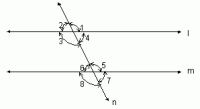kwizNET Subscribers, please login to turn off the Ads!
 Email us to get an instant 20% discount on highly effective K-12 Math & English kwizNET Programs!

#### Online Quiz (WorksheetABCD)

Questions Per Quiz = 2 4 6 8 10

### Grade 6 - Mathematics9.3 Angles Made by a Transversal With Two Parallel Lines - IIExample : In the above figure l//m; n is the transversal. If Đ1 = 110°, find the other angles ? Solution : Given that Đ1 = 110°. Đ1 =Đ5, since Đ1 and Đ5 are corresponding angles. Therefore Đ5 = 110° Đ5 = Đ3, since they are interior alternate angles. Therefore Đ3 = 110°. Đ3 = Đ 7, since they are corresponding angles. Therefore Đ7 = 110°. Đ4 + Đ5 = 180°, since Đ4 and Đ5 are the interior angles those are on the same side of the transversal. But Đ5 = 110° Therefore Đ4 = 180° - 110° = 70°. Đ4 = Đ6, since they are interior alternate angles. Therefore Đ6 = 70°. Đ2 = Đ6, since they are corresponding angles. Therefore Đ2 = 70°. Therefore Đ1 = 110° , Đ2 = 70°, Đ3 = 110°, Đ4 = 70°, Đ5 = 110°, Đ6 =70°, Đ7 = 110° and Đ8 = 70°. Directions:Answer the following questions. Also draw parallel lines with a transversal and show all the angles formed by them and write all the sets of congruent and supplementary angles.>
 Q 1: What is the sum of the measures of angle 2 and angle 7?180 degrees120 degrees150 degrees Q 2: What is the sum of the measures of angle 3 and angle 6?90 degrees180 degrees130 degrees Q 3: If angle 8 = 120 degrees, find angle 3.130 degrees120 degrees100 degrees Q 4: If angle 2 = 60 degrees, find angle 5.120 degrees80 degrees130 degrees Question 5: This question is available to subscribers only! Question 6: This question is available to subscribers only!

#### Subscription to kwizNET Learning System offers the following benefits:

• Unrestricted access to grade appropriate lessons, quizzes, & printable worksheets
• Instant scoring of online quizzes
• Progress tracking and award certificates to keep your student motivated
• Unlimited practice with auto-generated 'WIZ MATH' quizzes
• Child-friendly website with no advertisements
• Choice of Math, English, Science, & Social Studies Curriculums
• Excellent value for K-12 and ACT, SAT, & TOEFL Test Preparation
• Get discount offers by sending an email to discounts@kwiznet.com

 Quiz Timer